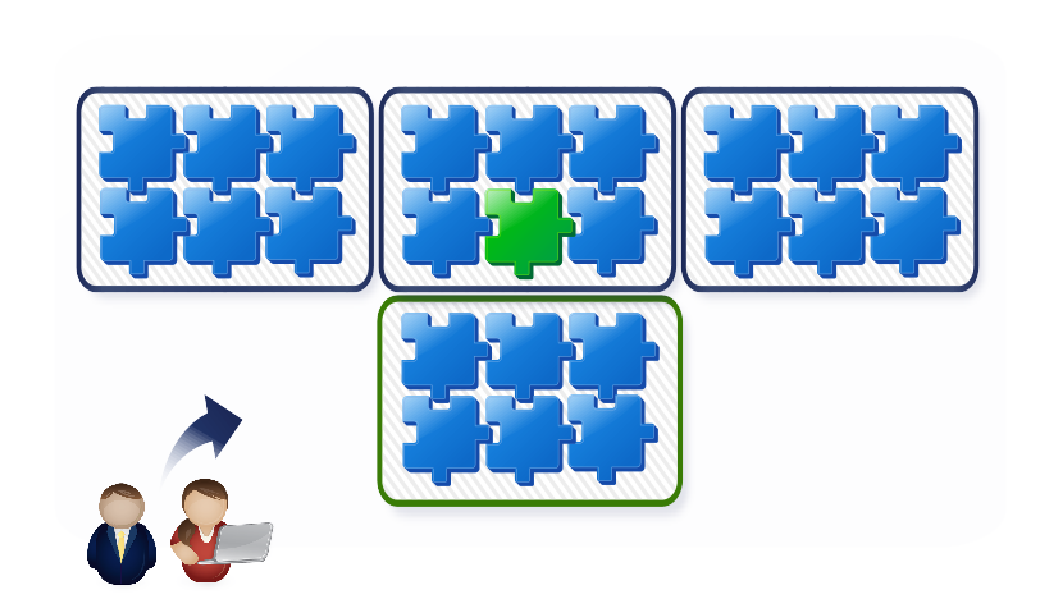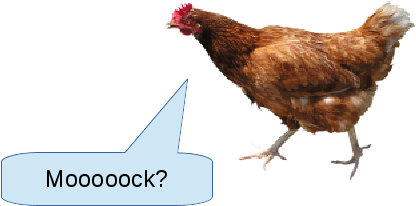## Mock Mock Mock

### Mocking-LibrariesClaudio Zizza

Developer for > 15 years
(Currently PHP)

Co-Organizer PHP Usergroup Karlsruhe

PHP Snippets: php.budgegeria.de

### Tests & Dependencies### DependenciesI only want to test/run
this small part

### Test Doubles

• Dummies
• Stubs
• Mocks
• Spy
(We do only Mocks for now)

### Tests are Confusing at some point### PHPUnit

``````
<?php

\$mockFw = new PHPUnit_Framework_MockObject_Generator();

\$math = \$mockFw->createMock(MathInterface::class);

\$math->expects(new PHPUnit_Framework_MockObject_Matcher_InvokedAtLeastOnce())
->method('sum')
->with(1, 1)
->willReturn(2);

\$math->__phpunit_verify();
``````

### Prophecy

``````
<?php

\$mockFw = new Prophet();

\$prophecy = \$mockFw->prophesize(MathInterface::class);
\$prophecy->sum(1, 1)
->willReturn(2)
->shouldBeCalledTimes(1);

\$math = \$prophecy->reveal();
``````

### Mockery

``````
<?php

\$math = Mockery::mock(MathInterface::class);
->once()
->with(1, 1)
->andReturn(2);

Mockery::close();
``````

### vfsStream### vfsStream

``````
<?php

baseDir = vfsStream::setup('dir');

mkdir(vfsStream::url('dir')  . '/' . 'myDir');
``````

### bovigo/callmap

``````
<?php

\$math = NewInstance::of(MathInterface::class)
->returns(['sum' => 2]);
// ...
verify(\$math, 'sum')->wasCalledOnce();
``````

### Phake

``````
<?php

\$math = Phake::mock(MathInterface::class);
Phake::when(\$math)->sum(1, 1)->thenReturn(2);
// ...
Phake::verify(\$math, Phake::times(1))->sum(1, 1);
``````

### PHP-Mock

``````
<?php

\$builder = new MockBuilder();
\$builder->setNamespace(__NAMESPACE__)
->setName('time')
->setFunction(function () {
return 0;
}
);
\$stub = \$builder->build();

\$stub->enable();
time();
``````

### AspectMock

``````
<?php

\$mathMock = Test::double(Math::class, ['sum' => 0]);

\$math = new Math();
\$testify->assertSame(0, \$math->sum(1, 2));

\$mathMock->verifyInvokedOnce('sum', [1, 2]);
``````

### Let's mock mock mock:

https://github.com/SenseException/mock-mock-mock

## Thank you

Claudio Zizza
php.budgegeria.de
@SenseException